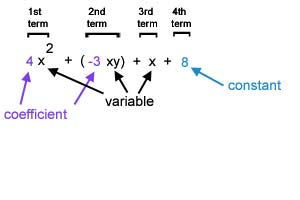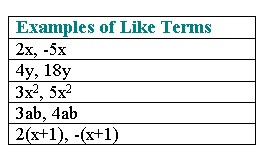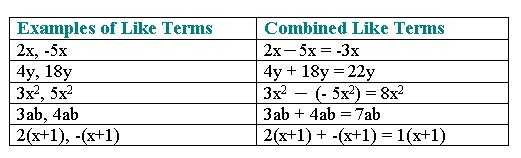Combine Like Terms

Combine Like Terms

Key Terms

A variable can represent a variety of numbers.

A number by itself, not directly affected by a variable, is called a constant .

An algebraic expression is a collection of numbers, variables, grouping symbols, and operation symbols. When an algebraic expression consists of several parts, each part that is added is called a term .

The numerical part of a term is called its numerical coefficient (or coefficient). In the term 5x, the 5 is the numerical coefficient. 5x means that the variable, x, is multiplied by 5.

Whenever a term appears without a numerical coefficient, we assume that the coefficient is 1. For example, x is the same as 1x, and -x is the same as -1x.Like Terms

Like terms (or similar terms) are terms that have the same variables with the same exponents. Constants, such as 4 or -3 are like terms because they are both numbers with a definite value. Only the coefficients may differ for terms to be categorized as like terms.Combining Like Terms

To combine like terms means to add or subtract the like terms in an expression.

1. Determine which terms are like terms.
2. Add or subtract the coefficients of the like terms.
3. Multiply the number found in step 2 by the common variable(s).Practice

1. Combine like terms in the algebraic expressions below.

a) 2x + 5x + 7

b) 2ab + 3a + 9

c) x + 3 + y - 1/2

d) x2 + 3x - 4x2

e) 12 + x - x2 - 7

f) 3ab + 3ab2 + 3a2b2 + 2ab2

g) 5y - y + 6y

2.  Combine like terms (simplify the expressions).

a) 3/5x - 2/3x

b) 6.47b - 8.2b

c) 3b + 5a - 4 - 2a

d) -2x2 + 4y - 3x2 + 2 - y + 5

1.)

a.) 7x + 7

b.) 2ab + 3a + 9

c.) x + 5/2 +

d.) -3x2 + 3x

e.) 5 + x - x2

f.) 3ab + 5ab2 + 3a2b2

g.) 10y

2.)

a.) (-1/15)x

b.) -1.73b

c.) 3a + 3b -4

d.) -5x2 + 3y + 7